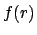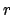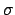Next: From Bayesian inference to Up: Inferring numerical values of Previous: Predictive distributions

## Hierarchical modelling and hyperparameters

As we have seen in the previous section, it is often desirable to include in a probabilistic model one's uncertainty in various aspects of a pdf. This is a natural feature of the Bayesian methods, due to the uniform approach to deal with uncertainty and from which powerful analysis tools are derived. This kind of this modelling is called hierarchical because the characteristics of one pdf are controlled by another pdf. All uncertain parameters from which the pdf depends are called hyperparameter. An example of use of hyperparameter is described in Sect. 8.3 in which the prior to inferin a binomial model are shown to be controlled by the parameters of a Beta distribution.

As an example of practical importance, think of the combination of experimental results in the presence of outliers, i.e. of data points which are somehow in mutual disagreement. In this case the combination rule given by Eqs. (30)-(32), extended to many data points, produces unacceptable conclusions. A way of solving the problem (Dose and von der Linden 1999, D'Agostini 1999b) is to model a scepticism about the quoted standard deviations of the experiments, introducing a pdf, whereis a rescaling factor of the standard deviation. In this way the's that enter the r.h.s. of Eqs. (30)-(32) are hyperparameters of the problem. An alternative approach, also based on hierarchical modelling, is shown in (Fröhner 2000). For a more complete introduction to the subject see e.g. (Gelman et al 1995).Next: From Bayesian inference to Up: Inferring numerical values of Previous: Predictive distributions
Giulio D'Agostini 2003-05-13